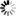Welcome to Central Library, SUST
Normal view

# Advances in Fuzzy Implication Functions [electronic resource] / edited by Michał Baczyński, Gleb Beliakov, Humberto Bustince Sola, Ana Pradera.

Material type:TextSeries: Studies in Fuzziness and Soft Computing ; 300Publisher: Berlin, Heidelberg : Springer Berlin Heidelberg : Imprint: Springer, 2013Description: VII, 209 p. online resourceContent type:
• text
Media type:
• computer
Carrier type:
• online resource
ISBN:
• 9783642356773
Additional physical formats: Printed edition:: No titleDDC classification:
• 006.3 23
LOC classification:
• Q342
Online resources:
Contents:
An Overview of Construction Methods of Fuzzy Implications -- Fuzzy Implications: Classification and a New Class -- A Survey of the Distributivity of Implications over Continuous T-norms and the Simultaneous Satisfaction of the Contrapositive Symmetry -- Implication Functions in Interval-valued Fuzzy Set Theory -- (S;N)-Implications on Bounded Lattices -- Implication Functions Generated Using Functions of one Variable -- Compositions of Fuzzy Implications -- Fuzzy Implications: Some Recently Solved Problems.
Summary: Fuzzy implication functions are one of the main operations in fuzzy logic. They generalize the classical implication, which takes values in the set {0,1}, to fuzzy logic, where the truth values belong to the unit interval [0,1]. These functions are not only fundamental for fuzzy logic systems, fuzzy control, approximate reasoning and expert systems, but they also play a significant role in mathematical fuzzy logic, in fuzzy mathematical morphology and image processing, in defining fuzzy subsethood measures and in solving fuzzy relational equations. This volume collects 8 research papers on fuzzy implication functions. Three articles focus on the construction methods, on different ways of generating new classes and on the common properties of implications and their dependencies. Two articles discuss implications defined on lattices, in particular implication functions in interval-valued fuzzy set theories. One paper summarizes the sufficient and necessary conditions of solutions for one distributivity equation of implication. The following paper analyzes compositions based on a binary operation * and discusses the dependencies between the algebraic properties of this operation and the induced sup-* composition. The last article discusses some open problems related to fuzzy implications, which have either been completely solved or those for which partial answers are known. These papers aim to present today’s state-of-the-art in this area.
Tags from this library: No tags from this library for this title.
Star ratingsAverage rating: 0.0 (0 votes)
No physical items for this record

An Overview of Construction Methods of Fuzzy Implications -- Fuzzy Implications: Classification and a New Class -- A Survey of the Distributivity of Implications over Continuous T-norms and the Simultaneous Satisfaction of the Contrapositive Symmetry -- Implication Functions in Interval-valued Fuzzy Set Theory -- (S;N)-Implications on Bounded Lattices -- Implication Functions Generated Using Functions of one Variable -- Compositions of Fuzzy Implications -- Fuzzy Implications: Some Recently Solved Problems.

Fuzzy implication functions are one of the main operations in fuzzy logic. They generalize the classical implication, which takes values in the set {0,1}, to fuzzy logic, where the truth values belong to the unit interval [0,1]. These functions are not only fundamental for fuzzy logic systems, fuzzy control, approximate reasoning and expert systems, but they also play a significant role in mathematical fuzzy logic, in fuzzy mathematical morphology and image processing, in defining fuzzy subsethood measures and in solving fuzzy relational equations. This volume collects 8 research papers on fuzzy implication functions. Three articles focus on the construction methods, on different ways of generating new classes and on the common properties of implications and their dependencies. Two articles discuss implications defined on lattices, in particular implication functions in interval-valued fuzzy set theories. One paper summarizes the sufficient and necessary conditions of solutions for one distributivity equation of implication. The following paper analyzes compositions based on a binary operation * and discusses the dependencies between the algebraic properties of this operation and the induced sup-* composition. The last article discusses some open problems related to fuzzy implications, which have either been completely solved or those for which partial answers are known. These papers aim to present today’s state-of-the-art in this area.

There are no comments on this title.